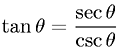Equations > Trigonometry > Trigonometric Properties > Quotient Property - Tangent, Secant, and Cosecant

Quotient Property - Tangent, Secant, and CosecantLatex Code:

MathML Code:

 $\mathrm{tan}\theta =\frac\mathrm{sec}\theta \mathrm{csc}\theta$

MathType 5.0: